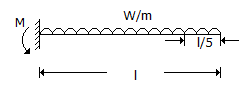# Civil Engineering - UPSC Civil Service Exam Questions

46.

If R is the radius of the main curve, θ the angle of deflection S the shift and L the length of the transition curve, then the total tangent length of the curve is given by

 A. (R - S) tan θ/2 - L/2 B. (R + S) tan θ/2 - L/2 C. (R + S) tan θ/2 + L/2 D. (R - S) tan θ/2 + L/2

Explanation:

No answer description available for this question. Let us discuss.

47.

Which one of the following, gives the correct decreasing order of the densities of a soil sample?

 A. Saturated, submerged, wet, dry B. Saturated, wet, submerged, dry C. Saturated, wet, dry, submerged D. Wet, saturated, submerged, dry

Explanation:

No answer description available for this question. Let us discuss.

48.

What does the critical path in PERT represent ?
1. The shortest path for the earliest completion of the project.
2. The longest path of the network from the initial to final event,

 A. 1 only B. 2 only C. Both 1 and 2 D. Neither 1 nor 2

Explanation:

No answer description available for this question. Let us discuss.

49.

A simply supported beam of constant width and varying depth and uniform strength is subjected to a central concentrated load. The depth of the beam dx at a distance x from one of the supports is proportional to

 A. x1/2 B. x1/3 C. x D. x2

Explanation:

No answer description available for this question. Let us discuss.

50.

In the given figure, the maximum bending moment at the fixed end of the cantilever caused by the UDL is M. The bending moment at section 1/5 from the free end isA. 4%of M B. 5% of M C. 10%of M D. 20% of M